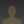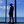0%
0%

# Autonomous Car: Neural Network + PID Controller

Machine learning and PID controller allow us to control an autonomous car to drive around an object

Similar projects worth following
135 views
1) MACHINE LEARNING: In this project we will create a NEURAL NETWORK with "Python" and copy its weights to a network with forward propagation on the "Artemis RedBoard ATP" board programmed with "Arduino IDE", and that will allow the robot car to drive alone and without hitting the walls. We will have four inputs, three correspond to 3 analog infrarred sensors and the fourth is for the BIAS. We will make the neural network have 4 outputs: two for each motor.

2) PID CONTROLLER: In this phase, also we will use the same 3 analog infrared sensors, which detect the distances at which the walls are, one in front and two on the left and right sides. The robot uses PID controller to maintain a central distance between the left and right walls. If the robot is near the left wall, then it can decrease the speed of the right motor and increase the speed of the left motor, to make the robot move to the right, and moving away from the left wall, and vice versa.

INTRODUCTION

The goals of this project are the following:

• Apply the machine learning to a robot car.
• Add the PID controller to this robot.

To facilitate the understanding of this project, we have divided it into the following sections: 1) Hardware, 2) Machine Learning, 3) PID Controller, 4) Printing and Assembling the Chassis, and 5) Conclusion.

1) HARDWARE

In the figure below I show you the schematic diagram.

SparkFun RedBoard Artemis

Features: Arduino Uno R3 Footprint, 1M Flash / 384k RAM, 48MHz / 96MHz turbo available, 24 GPIO - all interrupt capable, 21 PWM channels, Built in BLE radio, 10 ADC channels with 14-bit precision, 2 UARTs, 6 I2C buses, 4 SPI buses, PDM Interface, I2S Interface and Qwiic Connector.

2) MACHINE LEARNING

A very good machine learning bibliographic reference is the following: This post is in Spanish, and in my case it helped me to understand the theory and calculation of neural networks: https://www.aprendemachinelearning.com/crear-una-red-neuronal-en-python-desde-cero/

In this tutorial we will create a neural network with Python and copy its weights to a network with forward propagation on the Artemis RedBoard ATP board, and that will allow the robot car to drive alone and without hitting the walls.

For this exercise we will make the neural network have 4 outputs: two for each motor, since to the L298N driver we will connect 2 digital outputs of the board for each car motor. In addition the outputs will be between 0 and 1 (depolarize or polarize the motor).

We will have four inputs, three correspond to the 3 sensors and the fourth is for the BIAS, the values will be 0 and 1, and they are assigned with the following logic: The sensors on the left and right will have a value of 1 if the distance is less than 13 cm, and will have a value of 0 if the distance is greater than 13 cm. The center sensor will have a value of 1 if the distance is less than 16.7 cm, and will have a value of 0 if the distance is greater than 16.7 cm. The BIAS will have a value of 1. Here we see the changes in this table:

And the actions of the engines would be the following:

To create our neural network, we will use this code developed with Python 3.7.3: Neural_Network.py

```import numpy as np
# We create the class
class NeuralNetwork:
def __init__(self, layers, activation='tanh'):
if activation == 'sigmoid':
self.activation = sigmoid
elif activation == 'tanh':
self.activation = tanh
# Initialize the weights
self.weights = []
self.deltas = []
# Assign random values to input layer and hidden layer
for i in range(1, len(layers) - 1):
r = 2*np.random.random((layers[i-1] + 1, layers[i] + 1)) -1
self.weights.append(r)
# Assigned random to output layer
r = 2*np.random.random( (layers[i] + 1, layers[i+1])) - 1
self.weights.append(r)
def fit(self, X, y, learning_rate=0.2, epochs=100000):
# I add column of ones to the X inputs. With this we add the Bias unit to the input layer
ones = np.atleast_2d(np.ones(X.shape))
X = np.concatenate((ones.T, X), axis=1)
for k in range(epochs):
i = np.random.randint(X.shape)
a = [X[i]]
for l in range(len(self.weights)):
dot_value = np.dot(a[l], self.weights[l])
activation = self.activation(dot_value)
a.append(activation)
#Calculate the difference in the output layer and the value obtained
error = y[i] - a[-1]
deltas = [error * self.activation_prime(a[-1])]
# We start in the second layer until the last one (A layer before the output one)
for l in range(len(a) - 2, 0, -1):
deltas.append(deltas[-1].dot(self.weights[l].T)*self.activation_prime(a[l]))
self.deltas.append(deltas)
# Reverse
deltas.reverse()
# Backpropagation
# 1. Multiply the output delta with the input activations to obtain the weight gradient.
# 2. Updated the weight by subtracting a percentage of the gradient
for i in range(len(self.weights)):
layer...```

### chassis_up.stl

Secondary STL file of the chassis

Standard Tesselated Geometry - 11.61 kB - 05/19/2020 at 20:24

### chassis_1.stl

Main STL file of the chassis

Standard Tesselated Geometry - 32.41 kB - 05/19/2020 at 20:22

### microrobot_artemis.png

Schematic Diagram

Portable Network Graphics (PNG) - 1.08 MB - 05/19/2020 at 20:03

### Self_Driving_Car.ino

Code to test the neural network on the RedBoard Artemis ATP

ino - 5.62 kB - 05/19/2020 at 20:01

### Generate_Arduino_Code.py

Code to generate the coefficients of the Hidden weights and Output weights

py - 4.98 kB - 05/19/2020 at 19:59

• 1 × SparkFunn RedBoard Artimis ATP
• 1 × L298N Driver
• 1 × Rechargeable battery 7.2 V
• 3 × IR distance sensor
• 2 × Gearmotor
• 1
Notes:

Software apps and online services:

• Arduino IDE
• Python 3.7.3

Hand tools and fabrication machines:

• 3D Printer (generic)

Share

## Similar Projects

Project Owner Contributor

### OSCAR: Omni Service Cooperative Assistant RobotPoh Hou Shun

Project Owner Contributor

### Open-Source inverted pendulumTony-Lin

# Does this project spark your interest?

Become a member to follow this project and never miss any updates